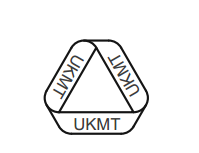# UKMT Senior Challenge-XVII

Algebra Level 2Given that $a+b=5$ and $ab=3$, what is the value of $a^4+b^4$?

This problem is not original.This problem is part of this set.

×